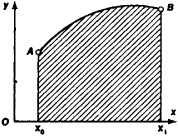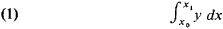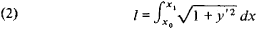# Isoperimetric Problems

Also found in: Dictionary.
The following article is from The Great Soviet Encyclopedia (1979). It might be outdated or ideologically biased.

## Isoperimetric Problems

a class of problems of the calculus of variations. The simplest isoperimetric problems (for example, finding triangles and polygons of given perimeter that have the greatest area; finding the closed curve of given length that bounds the maximum area; determining the closed surface of given area and maximum volume) were known to ancient Greek scholars (Archimedes, Zenodorus, and others).

The general study of isoperimetric problems was begun in 1697, when Jakob Bernoulli published an isoperimetric problem that he posed and partially solved: of all curves of given length, find the curve for which some quantity dependent on the curve reaches a minimum or maximum. A systematic study of isoperimetric problems was first made in 1732 by L. Euler. An example of an isoperimetric problem is: of curves of given length l passing through the points A and B, find the curve for which the areaFigure 1

of a curvilinear trapezoid (shaded in Figure 1) is greatest. The area of a curvilinear trapezoid is equal toand the length of the arc isConsequently, the problem reduces to finding the largest value of integral (1) under conditions (2). It turns out that the unknown curve is an arc of a circle.

### REFERENCE

Lavrent’ev, M. A., and L.A. Moscow-Leningrad, 1950.